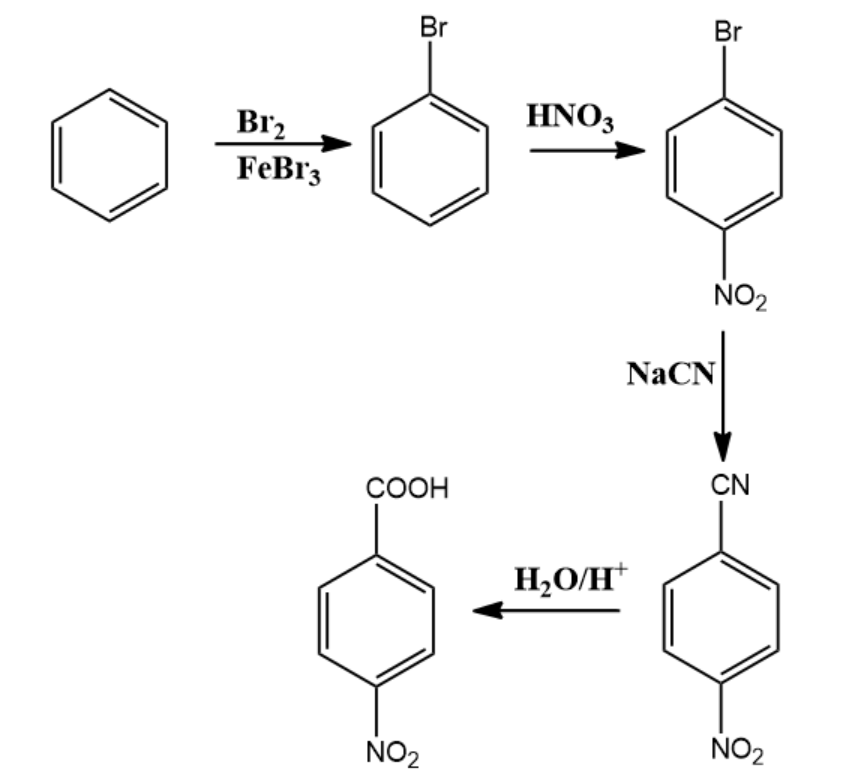Win up to 100% scholarship on Aakash BYJU'S JEE/NEET courses with ABNAT Win up to 100% scholarship on Aakash BYJU'S JEE/NEET courses with ABNAT

# JEE Main 2021 August 26 Shift 1 Chemistry Question Paper

JEE Main 2021 August 26 Shift 1 Chemistry question paper with solutions provided here will help students have a more effective preparation for the entrance exam. How? The JEE Main 2021 question paper for August 26 shift 1 will help students get an idea of the important topics from an exam point of view. Additionally, as candidates go through the questions they will get a better understanding of the format and types of questions, their difficulty level, weightage of marks and the overall exam pattern of JEE Main.

### JEE Main 2021 August 26 Shift 1 Chemistry Question Paper

Moreover, the solutions that are provided for each question will help JEE aspirants to get a good grip of the concepts and also remember the key steps to solve the problems. Besides, in this section questions are mostly concept based and having a thorough knowledge of the topics will help each student to perform better. JEE Main 2021 August 26 Shift 1 Chemistry question paper with solutions will do just that. This study material will aid students in studying productively for the exam. Students can view and download the JEE Main 2021 Chemistry question paper PDF for August 26 shift 1 below.

JEE Main 2021 August 26th Shift 1 Chemistry Question Paper

Question 1. Calculate the number of 4f electrons present in Gd.2+ (Atomic number = 64).

Electronic configuration of Gd = [Xe]4f75d1 6s2

Electronic configuration of Gd2+ = [Xe]4f75d1

Due to orbital contraction, the electron of 5d will get transferred into 4f and its electronic configuration will be Gd2+ = [Xe]4f85d0.

Hence, the number of 4f electrons present in

Gd2+ = 8

Question 2. Find the number of lone pairs on the central atom in interhalogen compound IX3

a. 1
c. 2
b. 3
d. 5

Hybridization of interhalogen compound IX3 = sp3d.

Structure: 3 bond pairs and 2 lone pairs, hence T shaped. Therefore, central atom I contains 2 lone pairs.

Hence, option c) is the correct answer.

Question 3. Below are two statements:

Statement I: Frenkel defect provides colour in a solid.

Statement II: Frenkel defect is an interstitial defect.

a. Statement I is correct, and statement II is correct.
b. Statement I is correct, and statement II is incorrect.
c. Statement I is incorrect, and statement II is incorrect.
d. Statement I is incorrect, and statement II is correct

Frenkel defect is shown by ionic solids. It is a dislocation defect. It is not interstitial

defect and it is not responsible to provide colour in the solid.

Hence, option c) is the correct answer.

Question 4. Formula of hydroxyapatite is:

a. (CaPO4)6.CaF2
b. 3[Ca3(PO4)2].Ca(OH)2
c. 3[Ca3(PO4)2].CaF2
d. Ca3(PO4)2.Ca(OH)2

Correct formula of hydroxyapatite is 3[Ca3(PO4)2].Ca(OH)2. Hydroxyapatite is the main component of phosphate rocks. Hence, b) is the correct answer.

a. Melamine
b. Bakelite
c. Polyester
d. None of the above

Novolac on heating with formaldehyde undergoes cross-linking to form an infusible solid mass called bakelite.

Hence, the correct option is b).

Question 6. Calculate the molarity of 3.3 molal solution of KCl whose density is 1.28 g/ mL.

a. 3.7 M
b. 5.0 M
c. 3.4 M
d. 2.5 M

Given,

Molality = 3.3 m

Density = 1.28 g / mL

By using formula,

Molality(m) = M×1000 / 1000 × d − M × Msolute

3.3 = M × 1000 / 1000 × 1.28 − M × 74.5

4224 − 245.85 M = 1000 M

1245.85 M = 4224

M = 4224 / 1245.85 = 3.39 ≈ 3.4 M

Hence, the correct option is c.

Question 7. The suitable method to deionize water is:

a. Synthetic resin
c. Permutit method
b. Calgon
d.Clark’s method

Pure demineralised (de-ionized) water free from all soluble mineral salts is obtained by passing water successively through a cation exchange (in the H+ form) and an anion exchange (in the OH form) resins.

Hence, the correct option is a.

Question 8. When an excess of CO2 is passed through lime water then what will be the sequence of the product?

a. Ca(HCO3)2,CaO
b. CaO,Ca(HCO3)2
c. CaCO3,Ca(HCO3)2
d. Ca(HCO3)2,CaO

Ca(OH)2 (aq) + CO2(g) → CaCO3(s) + H2O(l)

CaCO3(s) + H2O(l) + CO2(g) → Ca(HCO3)2(aq)

Hence, the correct option is c).

Question 9. Predict the major product for the following reaction: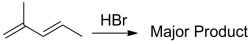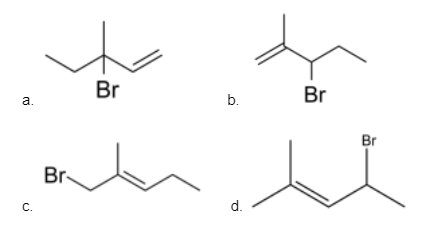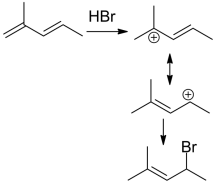Question 10. For adsorption of a gas:

a. ΔH < 0, ΔS < 0
b. ΔH < 0, ΔS > 0
c. ΔH > 0, ΔS > 0
d. ΔH = 0, ΔS > 0

During adsorption, there is always a decrease in residual forces of the surface, i.e.,

there is a decrease in surface energy which appears as heat. Adsorption, therefore, is

invariably an exothermic process, i.e., ΔH < 0. When a gas is adsorbed, the freedom

of movement of its molecules becomes restricted. This amounts to a decrease in the

entropy of the gas after adsorption, i.e., ΔS < 0 is negative.

Hence, the correct option is a.

Question 11. Find the product.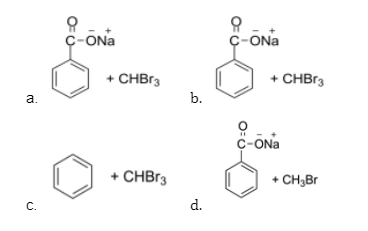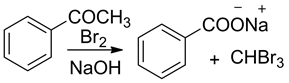Question 12. (A) and (B) respectively will be: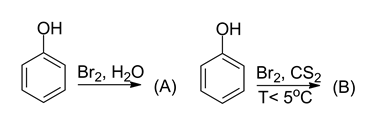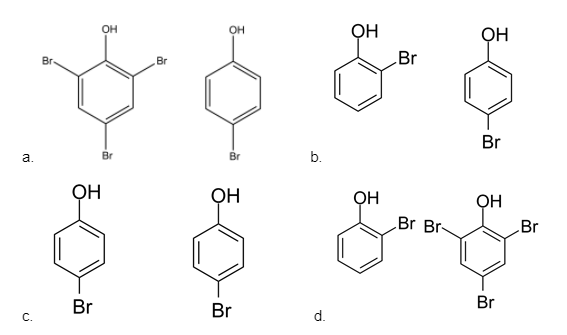In water, bromine is ionized to a greater extent and hence tri bromination occurs. In

Carbon disulphide, bromine ionizes to a very less extent.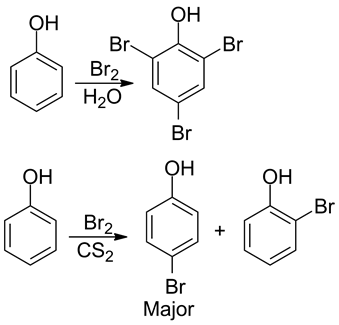Question 13. Choose the ion whose aqueous solution is violet coloured?

a. [FeCN6]4-
b. [Fe(SCN)3]
c. [Fe(CN)5NOS]4-
d. [Fe(CN)6]3-

Violet colour is given by complex ions [𝐹𝑒(𝐶𝑁)5𝑁𝑂𝑆]4-. It is the test for the detection of sulphur.

Question 14. Choose the correct option for the statements given below.

Statement-I: Ellingham diagram is used to check which metal compound is to be reduced by which reducing agent.

Statement-II: In the Ellingham diagram, as we move from left to right[Equation] increases.

a. Both S-I and S-II are true
b. S-I is true, but S-II is false
c. S-I is false, but S-II is false
d. Both S-I and S-II are false

S1: Ellingham diagram is used to check which metal oxide is to be reduced by which compound.

Explanation: This statement is true because the graph of metal that lies below in the Ellingham diagram has more affinity towards oxygen. Therefore, it can be used to predict which metal oxide can be reduced by using a particular compound.

S2: In the Ellingham diagram, as we move from left to right[Equation] increases.

Explanation: This statement is false because the slope of the Ellingham diagram is equal to −∆S and the Ellingham diagram contains a straight line for which slope is constant. It gets changed only when the phase is changed.

Hence, option b is correct

Question 15. Which compound will have an equal freezing point as 0.1 Methanol?

a. 0.1 𝑀 𝑁𝑎2𝑆𝑂4
b. 0.1 𝑀 𝐵𝑎3(𝑃𝑂4)2
c. 0.1 𝑀 𝐻𝐶𝑙
d. 0.1 𝑀 𝐶6𝐻12𝑂6

Δ𝑇𝑓 = 𝑖𝐾𝑓𝑚

For very dilute solutions, we can assume 𝑚(𝑚𝑜𝑙𝑎𝑙𝑖𝑡𝑦) = 𝑀(𝑀𝑜𝑙𝑎𝑟𝑖𝑡𝑦)

As m is the same for all solutions so Δ𝑇𝑓 depends on the vant Hoff factor (i).

Greater is the i, smaller will be the freezing point.

Let’s compute for each option:

a. 𝑖 𝑓𝑜𝑟 0.1 𝑀 𝑁𝑎2𝑆𝑂4 = 3

b. 𝑖 𝑓𝑜𝑟 0.1 𝑀 𝐵𝑎3(𝑃𝑂4)2 = 5

c. 𝑖 𝑓𝑜𝑟 0.1 𝑀 𝐻𝐶𝑙 = 2

d. 𝑖 𝑓𝑜𝑟 0.1 𝑀 𝐶6𝐻12𝑂6 = 1

And i for ethanol (𝐶2𝐻5𝑂𝐻) = is also 1.

Hence, the correct answer will be d.

Question 16. Which of the following will dissolve in water and give colour?

a. 𝐶𝑢2𝐶𝑙2
b. 𝐶𝑢𝐶𝑙2
c. 𝐴𝑔𝐵𝑟
d. 𝑍𝑛𝐶𝑙2

𝐶𝑢𝐶𝑙2 dissolves in water and gives coloured solutions.

Question 17. Choose the correct option for the below-given statements.

Statement I: In Bohr’s model, the velocity of electrons increases with a decrease in the positive charge of the nucleus as electrons are not held tightly.

Statement II: Velocity decreases with an increase in principal quantum number.

a. Statement I is correct & Statement II is correct
b. Statement I is correct & Statement II is incorrect
c. Statement I is incorrect & Statement II is incorrect
d. Statement I is incorrect & Statement II is correct

For Statement I:

According to Bohr’s model,

Velocity of electron,

$$\begin{array}{l}V_{n} = v_{o}\tfrac{z}{n}\end{array}$$

Where, 𝑣o = 2.18 × 106 𝑚/𝑠

So, with a decrease in z, Vn will also decrease

Hence, Statement I is incorrect,

For Statement II:

With an increase in n, velocity will decrease as per the relation given above,

Hence, Statement II is correct

Hence, option d) is correct.

Question 18. Correct sequence of [Equation] of [Equation] at constant temperature.

a.

$$\begin{array}{l}U_{N_{2}} > U_{O_{2}} > U_{CO_{2}}\end{array}$$

b.
$$\begin{array}{l}U_{O_{2}} > U_{N_{2}} > U_{CO_{2}}\end{array}$$

c.
$$\begin{array}{l}U_{CO_{2}} > U_{O_{2}} > U_{N_{2}}\end{array}$$

d.
$$\begin{array}{l}U_{CO_{2}} = U_{O_{2}} = U_{N_{2}}\end{array}$$

$$\begin{array}{l}U_{rms} = \sqrt{\frac{3RT}{M}}\end{array}$$

So,

$$\begin{array}{l}U_{rms}\: \alpha\: \frac{1}{\sqrt{m}}\end{array}$$
(At constant temperature)

And as we know, Molar Mass: CO2 > 𝑂2 > N2

Therefore, 𝑈rms ∶ 𝑈𝑁2 > 𝑈𝑂2 > 𝑈𝐶𝑂2

Hence, option a) is correct.

Question 19. Choose the correct option for the given statements.

Statement-I: Titration of a strong acid with a weak base uses methyl orange as an indicator.

Statement-II: Titration of a weak acid with a strong base uses phenolphthalein as an indicator.

a. Statement I is correct and Statement II is correct
b. Statement I is correct and Statement II is incorrect
c. Statement I is incorrect and Statement II is incorrect
d. Statement I is incorrect and Statement II is correct

Statement I: Methyl Orange is a suitable indicator for titration of strong acid and weak base is correct.

Statement II: Phenolphthalein is a suitable indicator for titration of a weak acid and a strong base is also correct.

Hence, option a) is correct.

Question 20. In the given equation:

𝐼𝑠𝑜𝑏𝑢𝑡𝑎𝑛𝑒 + 𝐵𝑟2(ℎ𝑣 / 𝑡𝑒𝑚𝑝 = 125o𝐶) → ?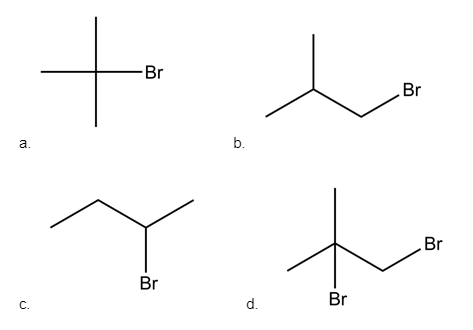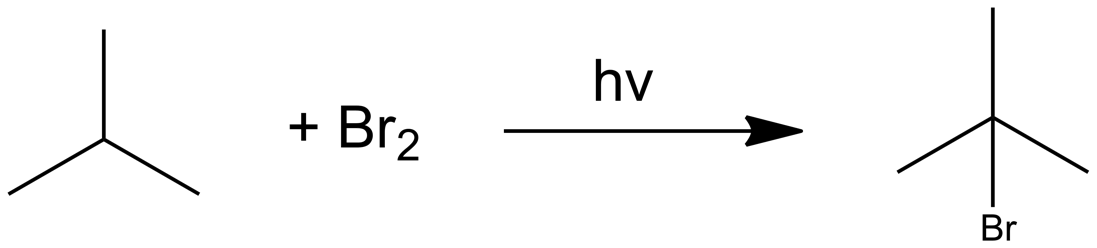Bromination is highly selective.

Reactivity order is: 3o > 2o > 1o.

Question 21. The ratio of water of crystallization present in Mohr’s salt to that of Potash alum is……..x 10-1?

Potash alum = K2(SO4).Al2(SO4)3.24H2O

Mohr’s salt = (NH4)2Fe(SO4)2.6H2O

Ratio of water molecule =

$$\begin{array}{l}\frac{6(From \: Mohr’s\: Salt)}{24(From \: Potash\: Alum)}\end{array}$$
= 0.25 = 2.5 x 10-1

Hence, the correct answer is 2.5

Question 22. Which of the following is the correct sequence of the following conversion?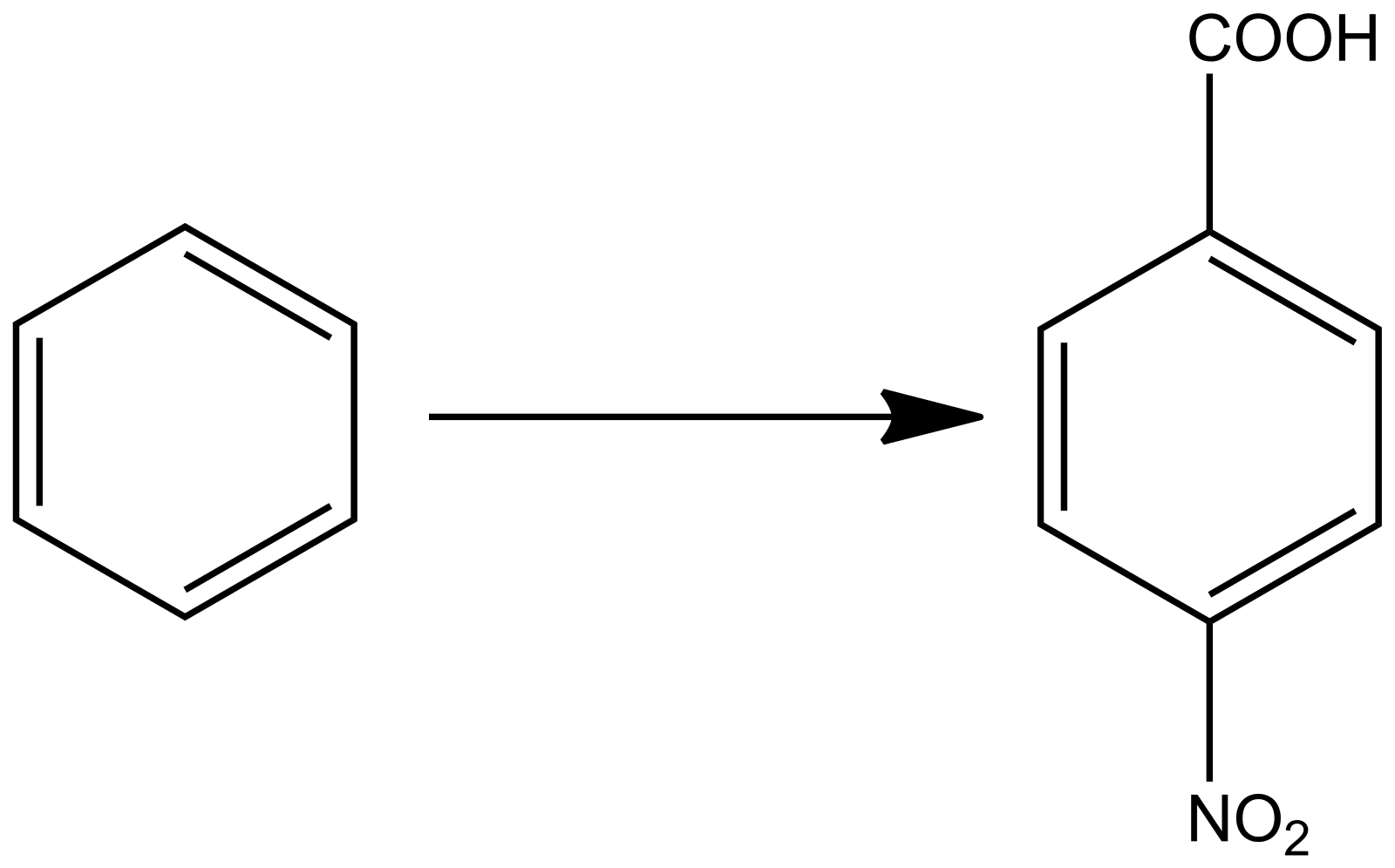a. Br2 / FeBr3, HNO3 / H2SO4, NaCN, H2O / H+
b. HNO3 / H2SO4, Br2 / FeBr3, Mg / Ether, CO2, H3O+
c. NaCN, H3O+, Br2 / FeBr3, H3O+ / CO2 / H2O
d. HNO3 / H2SO4, Br2 / FeBr3 / NaCN/H2O